# Similarity Metrics in NLP

This post covers the use of euclidean distance, dot product, and cosine similarity as NLP similarity metrics.

By James Briggs, Data ScientistImage by the author

When we convert language into a machine-readable format, the standard approach is to use dense vectors.

A neural network typically generates dense vectors. They allow us to convert words and sentences into high-dimensional vectors — organized so that each vector's geometric position can attribute meaning.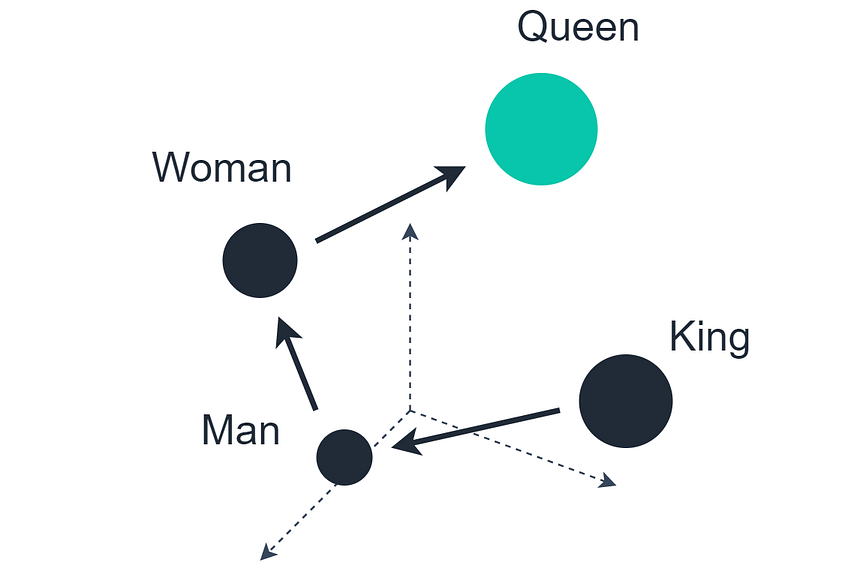The well-known language arithmetic example showing that Queen = King — Man + Woman

There is a particularly well-known example of this, where we take the vector of King, subtract the vector Man, and add the vector Woman. The closest matching vector to the resultant vector is Queen.

We can apply the same logic to longer sequences, too, like sentences or paragraphs — and we will find that similar meaning corresponds with proximity/orientation between those vectors.

So, similarity is important — and what we will cover here are the three most popular metrics for calculating that similarity.

### Euclidean Distance

Euclidean distance (often called L2 norm) is the most intuitive of the metrics. Let’s define three vectors: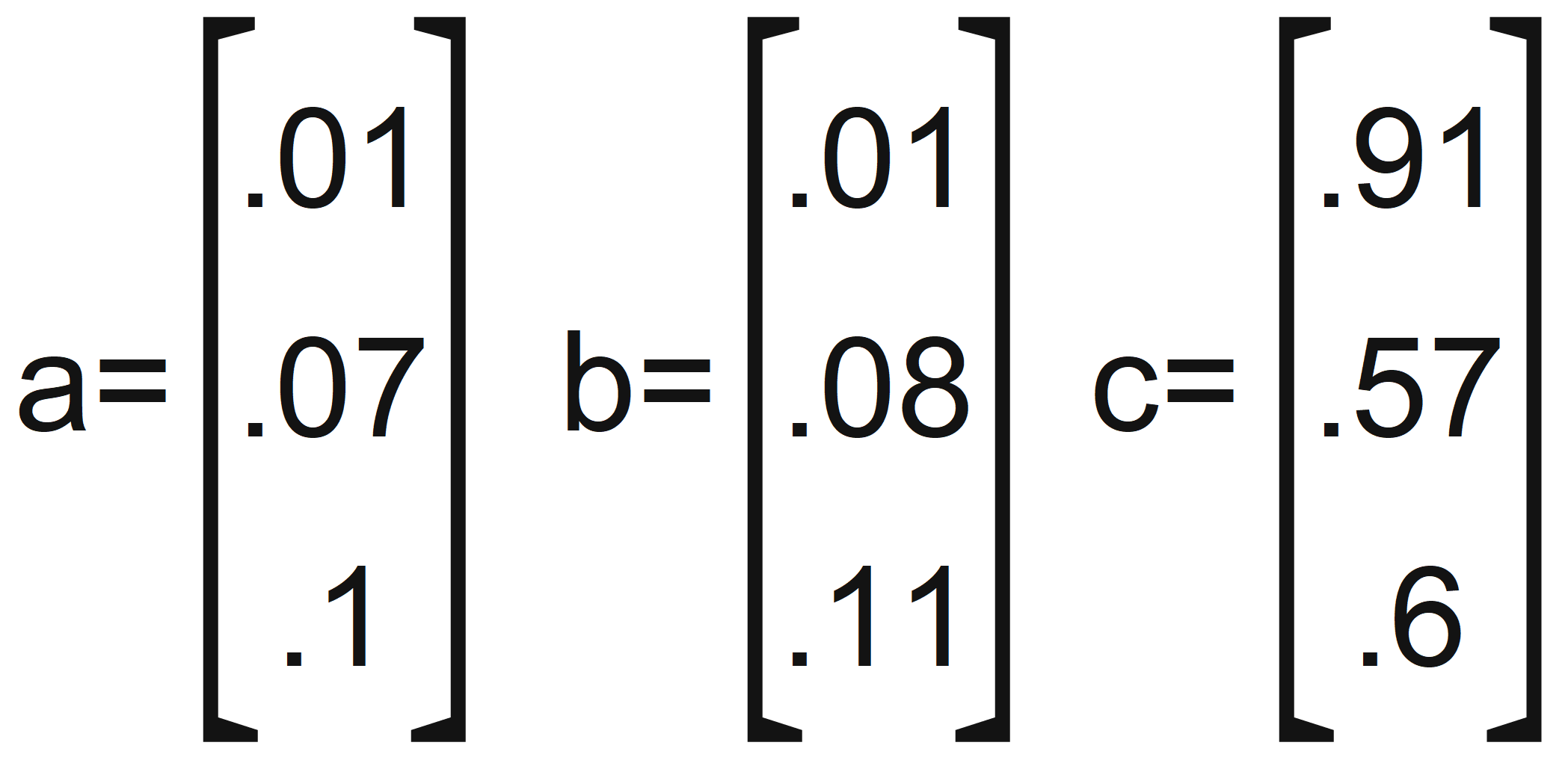Three vector examples

Just by looking at these vectors, we can confidently say that a and b are nearer to each other — and we see this even clearer when visualizing each on a chart: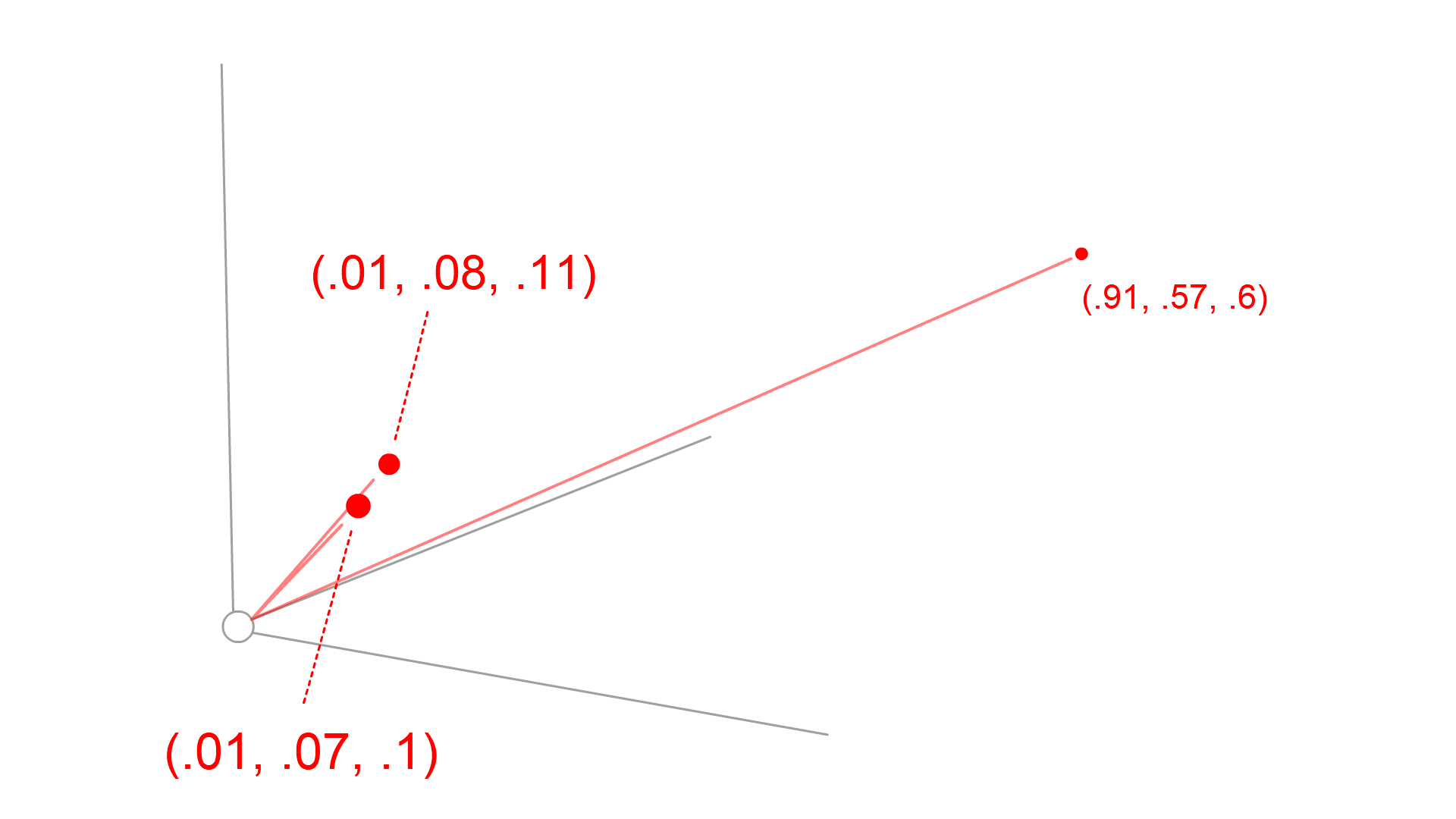Vectors a and b are close to the origin, vector c is much more distant

Clearly, a and b are closer together — and we calculate that using Euclidean distance:Euclidean distance formula

To apply this formula to our two vectors, a and b, we do: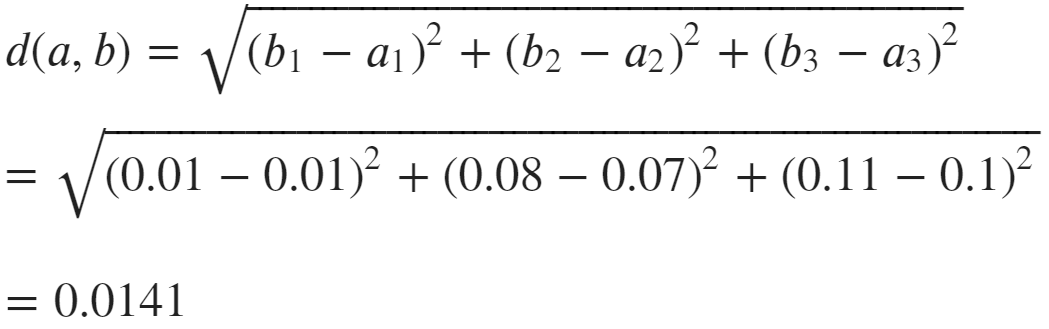Calculation of Euclidean distance between vectors a and b

And we get a distance of 0.014, performing the same calculation for d(a, c) returns 1.145, and d(b, c) returns 1.136. Clearly, a and b are nearer in Euclidean space.

### Dot Product

One drawback of Euclidean distance is the lack of orientation considered in the calculation — it is based solely on magnitude. And this is where we can use our other two metrics. The first of those is the dot product.

The dot product considers direction (orientation) and also scales with vector magnitude.

We care about orientation because similar meaning (as we will often find) can be represented by the direction of the vector — not necessarily the magnitude of it.

For example, we may find that our vector's magnitude correlates with the frequency of a word that it represents in our dataset. Now, the word hi means the same as hello, and this may not be represented if our training data contained the word hi 1000 times and hello just twice.

So, vectors' orientation is often seen as being just as important (if not more so) as distance.

The dot product is calculated using:Dot product formula

The dot product considers the angle between vectors, where the angle is ~0, the cosθ component of the formula equals ~1. If the angle is nearer to 180 (orthogonal/perpendicular), the cosθ component equals ~0.

Therefore, the cosθ component increases the result where there is less of an angle between the two vectors. So, a higher dot-product correlates with higher orientation.

Again, let’s apply this formula to our two vectors, a and b: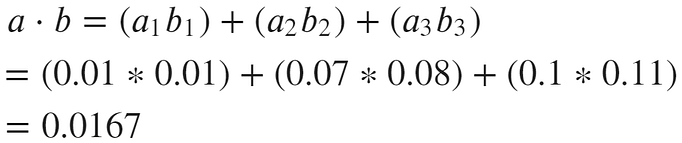Calculation of dot product for vectors a and b

Clearly, the dot product calculation is straightforward (the simplest of the three) — and this gives us benefits in terms of computation time.

However, there is one drawback. It is not normalized — meaning larger vectors will tend to score higher dot products, despite being less similar.

For example, if we calculate a·a — we would expect a higher score than a·c (a is an exact match to a). But that’s not how it works, unfortunately.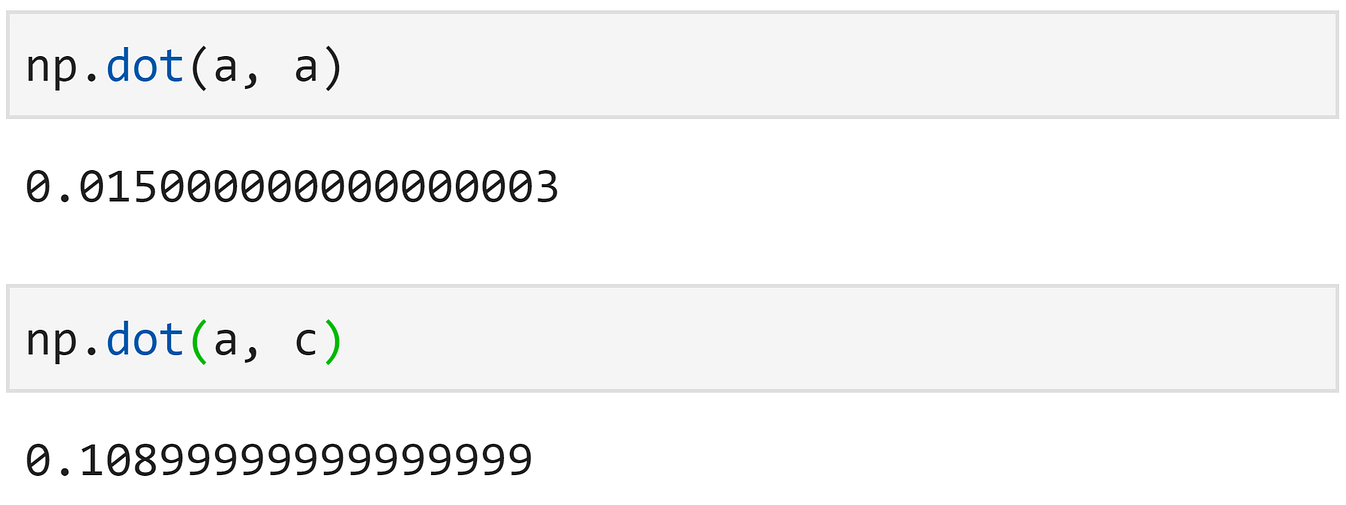The dot product isn’t so great when our vectors have differing magnitudes.

So, in reality, the dot-product is used to identify the general orientation of two vectors — because:

• Two vectors that point in a similar direction return a positive dot-product.
• Two perpendicular vectors return a dot-product of zero.
• Vectors that point in opposing directions return a negative dot-product.

### Cosine Similarity

Cosine similarity considers vector orientation, independent of vector magnitude.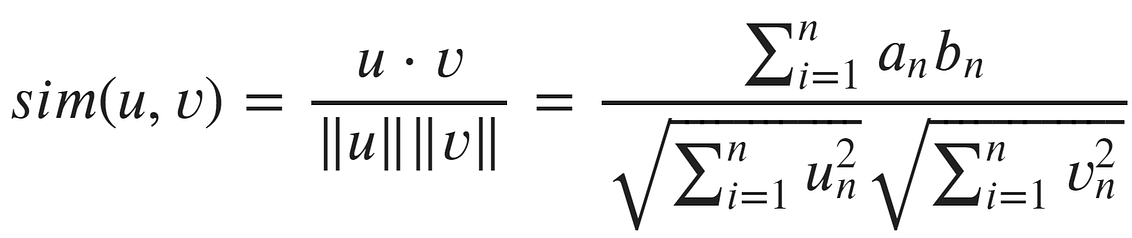Cosine similarity formula

The first thing we should be aware of in this formula is that the numerator is, in fact, the dot product — which considers both magnitude and direction.

In the denominator, we have the strange double vertical bars — these mean ‘the length of’. So, we have the length of u multiplied by the length of v. The length, of course, considers magnitude.

When we take a function that considers both magnitude and direction and divide that by a function that considers just magnitude — those two magnitudes cancel out, leaving us with a function that considers direction independent of magnitude.

We can think of cosine similarity as a normalized dot product! And it clearly works. The cosine similarity of a and b is near 1 (perfect):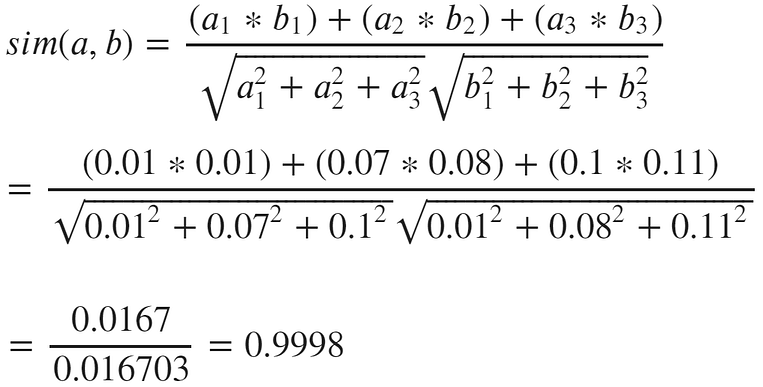Calculation of cosine similarity for vectors a and b

And using the `sklearn` implementation of cosine similarity to compare a and c again gives us much better results: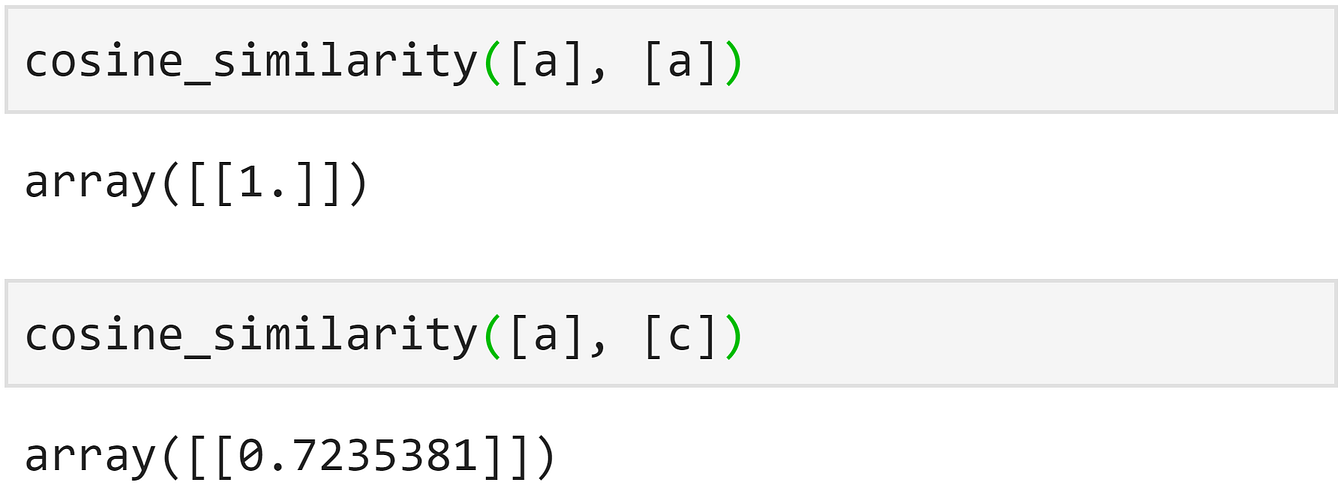Cosine similarity can often provide much better results than the dot product.

That’s all for this article covering the three distance/similarity metrics — Euclidean distance, dot product, and cosine similarity.

It’s worth being aware of how each works and their pros and cons — as they’re all used heavily in machine learning, and particularly NLP.

You can find Python implementations of each metric in this notebook.

I hope you’ve enjoyed the article. Let me know if you have any questions or suggestions via Twitter or in the comments below. If you’re interested in more content like this, I post on YouTube too.

*All images are by the author except where stated otherwise

Bio: James Briggs is a data scientist specializing in natural language processing and working in the finance sector, based in London, UK. He is also a freelance mentor, writer, and content creator. You can reach the author via email (jamescalam94@gmail.com).

Original. Reposted with permission.

Related: